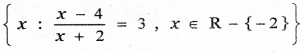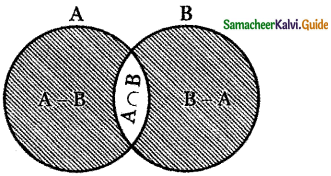Tamilnadu State Board New Syllabus Samacheer Kalvi 11th Maths Guide Pdf Chapter 1 Sets, Relations and Functions Ex 1.1 Text Book Back Questions and Answers, Notes.

## Tamilnadu Samacheer Kalvi 11th Maths Solutions Chapter 1 Sets, Relations and Functions Ex 1.1

Question 1.
Write the following in roaster form.
(i) {x ∈ N : x2 < 121 and x is a prime}
Let A = { x ∈ N : x2 < 121 and x is a prime }
A = { 2, 3, 5, 7 }(ii) The set of positive roots of the equation (x – 1) ( x + 1) (x – 1 ) = 0
The set of positive roots of the equations
(x – 1) (x + 1) (x2 – 1) = 0
(x – 1 ) (x + 1 ) (x + 1) (x – 1) = 0
(x + 1 )2 (x – 1)2 = 0
(x + 1)2 = 0 or (x – 1)2 = 0
x + 1 = 0 or x – 1 = 0
x = -1 or x = 1
A = { 1 }

(iii) {x ∈ N : 4x + 9 < 52}
4x + 9 < 52
4x + 9 – 9 < 52 – 9
4x < 43
x < $$\frac{43}{4}$$ (i.e.) x < 10.75 4
But x ∈ N
∴ A = {1, 2, 3, 4, 5, 6, 7, 8, 9, 10}(iv)Let A =⇒ $$\frac{x-4}{x+2}$$ = 3
⇒ x – 4 = 3(x + 2)
⇒ x – 4 = 3x + 6
⇒ 3x – x = – 4 – 6
2x = – 10
⇒ x = $$-\frac{10}{2}$$ = -5
A = { -5 }

Question 2.
Write the set {-1, 1} in set builder form.
A = {x : x2 – 1 = 0, x ∈ R}

Question 3.
State whether the following sets are finite or infinite.
(i) {x ∈ N : x is an even prime number }
Let A = { x ∈ N : x is an even prime number )
A = {2}
A is a finite set.

(ii) {x ∈ N: x is an odd prime number }
Let B = {x ∈ N : x is an odd prime number}
B = {1, 3, 5, 7, 11, …………….. }
B is an infinite set.(iii) {x ∈ Z : x is even and < 10 }
C = {x ∈ Z : x is even and< 10}
C = { ……….. -8, -6, -4, -2, 0, 2, 4, 6, 8}
C is an infinite set.

(iv) {x ∈ R : x is a rational number }
D = { x ∈ R : x is a rational number }
D is an infinite set.

(v) {x ∈ N: x is a rational number }
E = { x ∈ N : x is a rational number )
E = {1, 2, 3, 4, 5, 6, …………..)
Every integer is a rational number.
∴ E is an infinite set.Question 4.
By taking suitable sets A, B, C, verify the following results.
(i) A × (B ∩ C) = (A × B) ∩ (A × C)
To prove: A × (B ∩ C) = (A × B) ∩ (A × C)
B ∩ C = {8}; A = {1, 2, 5, 7}
So A × (B ∩ C) = {1, 2, 5, 7} × {8}
= {(1, 8), (2. 8), (5, 8), (7, 8)}
Now A x B = {(1, 2), (1, 7), (1, 8), (1, 9), (2, 2), (2, 7), (2, 8), (2, 9), (5, 2), (5, 7), (5, 8), (5, 9), (7, 2), (7, 7), (7, 8), (7, 9)} …. ( 1)
A × C = {(1, 1), (1, 5),(1, 8), (1, 10), (2, 1), (2, 5), (2, 8), (2, 10), (5, 1), (5, 5), (5, 8), (5, 10), (7, 1), (7, 5), (7, 8), (7, 10)}
(A × B) ∩ (A × C) = {(1, 8), (2, 8), (5, 8), (7, 8)} ……… (2)
(1) = (2)
⇒ A × (B ∩ C) = (A × B) ∩ (A × C)

(ii) A × (B ∪ C) = (A × B) ∪ (A × C)
Let A = {1, 2} , B = {3, 4}, C = {4, 5}
B ∪ C = {3, 4} ∪ {4, 5}
B ∪ C = {3, 4, 5)
A × (B ∪ C) = {1, 2} × {3, 4, 5}
A × (B ∪ C) = { (1, 3),( 1, 4),(1, 5),(2, 3), (2, 4),(2,5)} ——– (1)
A × B = {1, 2} × {3, 4}
A × B = { (1, 3), (1, 4), (2, 3), (2, 4) }
A × C = {1, 2} × {4, 5}
A × C = { (1, 4), (1, 5), (2, 4), (2, 5 )}
(A × B) ∪ (A × C) = {(1, 3), (1, 4), (2, 3), (2, 4)} ∪ {(1, 4 ), (1, 5 ), ( 2, 4 ), (2, 5)}
(A × B) ∪ (A × C) = { (1, 3) (1, 4), (1, 5), (2, 3), (2, 4), (2, 5)} —— (2)
From equations (1) and (2)
A × (B U C) = (A × B) U (A × C)(iii) (A × B) ∩ (B × A) = (A ∩ B) × ( B ∩ A)
A × B = {(1, 2), (1, 7), (1, 8), (1, 9) (2, 2), (2, 7), (2, 8), (2, 9) (5, 2), (5, 7), (5, 8), (5, 9) (7, 2), (7, 7), (7, 8), (7, 9)}
B × A = {(2, 1), (2, 2), (2, 5), (2, 7) (7, 1), (7, 2), (7, 5), (7, 7) (8, 1), (8, 2), (8, 5), (8, 7) (9,1), (9, 2), (9, 5), (9, 7)}
L.H.S. (A × B) ∩ (B × A) = {(2, 2), (2, 7), (7, 2), (7, 7)} …. (1)
R.H.S. A ∩ B = {2, 7}
B ∩ A = {2, 7}
(A ∩ B) × (B ∩ A) = {2, 7} × {2, 7}
= {(2, 2), (2, 7), (7, 2), (7, 7)} ……… (2)
(1) = (2) ⇒ LHS = RHS

(iv) C – (B – A) = (C ∩ A) ∪ (C ∩ B’)
Let A = {1, 2, 3) , B = {2, 3, 4) , C = {3, 4, 5}B – A = { 2, 3, 4 ) – {1, 2, 3}
B – A = {4}
C – (B – A) = {3, 4, 5} – {4}
C – (B – A) = {3, 5} —- (1)
C ∩ A = {3, 4, 5} ∩ { 1, 2, 3)
C ∩ A = {3}
B’ = {1, 5}
C ∩ B’ = {3, 4, 5} ∩ {1, 5}
C ∩ B’ = {5}
(C ∩ A) ∪ (C∩B’) = {3} ∪ {5}
(C∩A) ∪ (C ∩ B’) = {3, 5} —— (2)
From equations (1) and (2)
C – (B – A) = (C ∩ A) u (C ∩ B’)(v) (B – A) ∩ C = (B ∩ C) – A = B ∩ (C – A)
To prove (B – A) ∩ C = (B ∩ C) – A = B ∩ (C – A)
A= {1, 2, 5, 7}, B = {2, 7, 8, 9}, C = {1, 5, 8, 10}
Now B – A = {8, 9}
(B – A) ∩ C = {8} ……. (1)
B ∩ C = {8}
A = {1, 2, 5, 7}
So (B ∩ C) – A = {8} …… (2)
C – A = {8, 10}
B = {2, 7, 8, 9}
B ∩ (C – A) = {8} …. (3)
(1) = (2) = (3)

(vi) (B – A) ∪ C = (B ∪ C) – (A – C)
Let A = {1, 2, 3, 4}, B = {3, 4, 5, 6}, C = {5, 6, 7, 8}
B – A = {3, 4, 5, 6} – {1, 2, 3, 4}
B – A = {5, 6}
(B – A) ∪ C = {5, 6} ∪ {5, 6, 7, 8}
(B – A) ∪ C = { 5 , 6 , 7 , 8 } ——- (1)
B ∪ C = { 3, 4, 5, 6 } ∪ { 5, 6, 7,8 }
B ∪ C = { 3, 4, 5, 6, 7, 8 }
A – C = { 1, 2, 3, 4 } – { 5, 6, 7, 8 }
A – C = { 1 , 2, 3 , 4 }
(B ∪ C) – (A – C) = {3, 4, 5, 6, 7, 8} – {1, 2, 3, 4}
(B ∪ C) – (A – C) = { 5, 6, 7, 8 } —-(2)
From equations (1) and (2)
(B – A) ∪ C = (B ∪ C) – (A – C)Question 5.
Justify the trueness of the statement: “An element of a set can never be a subset of itself”.
A set itself can be a subset of itself (i.e.) A ⊆ A.
But it cannot be a proper subset.

Question 6.
If n(P(A)) = 1024, n(A ∪ B) = 15 and n(P(B)) = 32, find n(A ∩ B).
Given n(P(A)) = 1024 , n(A ∪ B) = 15, n(P(B)) = 32
n(P(A)) = 1024 = 210 n(A) = 10
n(P(B)) = 32 = 25 = n(B) = 5
n(A ∪ B) = n(A) + n(B) – n(A ∩ B)
15 = 10 + 5 – n(A ∩ B)
15 = 15 – n (A ∩ B)
n(A ∩ B) = 0

Question 7.
If n (A ∩ B ) = 3 and n(A ∪ B ) = 10 , then find n(P(A ∆ B)).n(A ∪ B) = 10; n(A ∩ B) = 3
n(A ∆ B) = 10 – 3 = 7
and n(P(A ∆ B)) = 27 = 128Question 8.
For a set A, A × A contains 16 elements and two of its elements are (1, 3) and (0, 2). Find the elements of A.
Given A × A contains 16 elements.
∴ A contains 4 elements.
Also, (1, 3) and (0, 2) are two elements of A × A.
∴ A = { 0, 1, 2, 3 }

Question 9.
Let A and B be two sets such that n (A) = 3 and n(B) = 2. If (x, 1), (y, 2), (z, 1) are in A × B , find A and B, where x , y , z are distinct elements.
n(A) = 3 ⇒ set A contains 3 elements
n(B) = 2 ⇒ set B contains 2 elements –
we are given (x, 1), (y, 2), (z, 1) are elements in A × B ⇒ A = {x, y, z} and B = {1, 2}Question 10.
If A × A has 16 elements, S = { ( a, b ) ∈ A × A: a < b } ; (-1, 2) and (0, 1) are two elements of S , then find the remaining elements of S.